• rnn公式
千次阅读
2018-04-22 16:03:44

## 通过时间反向传播

t=1 t = 1 $t=1$ t=τ t = τ $t=\tau$ 应用如下更新方程:

a(t)=b+Wh(t1)+Ux(t) a ( t ) = b + W h ( t − 1 ) + U x ( t ) $a^{(t)} = b + Wh^{(t-1)} + Ux^{(t)}$

h(t)=tanh(a(t)) h ( t ) = t a n h ( a ( t ) ) $h^{(t)} = tanh(a^{(t)})$

o(t)=c+Vh(t) o ( t ) = c + V h ( t ) $o^{(t)} = c + Vh^{(t)}$

y^(t)=softmax(o(t)) y ^ ( t ) = s o f t m a x ( o ( t ) ) $\hat y^{(t)} = softmax(o^{(t)})$

设损失函数 L(t) L ( t ) $L^{(t)}$为给定 x(1),...,x(τ) x ( 1 ) , . . . , x ( τ ) $x^{(1)}, ..., x^{(\tau)}$ y(t) y ( t ) $y^{(t)}$的负对数似然，则:

L(x,y)=tlogpmodel(y(t)|{x(1),...,x(τ)}) L ( x , y ) = − ∑ t l o g p m o d e l ( y ( t ) | { x ( 1 ) , . . . , x ( τ ) } ) $L(x,y) = - \sum_{t} log p_{model}( y^{(t)} | \left \{ x^{(1)}, ..., x^{(\tau)} \right \} )$

=logexp(o(t)y(t))iexp(o(t)i) = − l o g e x p ( o y ( t ) ( t ) ) ∑ i e x p ( o i ( t ) ) $= -log \frac{exp( o^{(t)}_{y(t)} )}{\sum_i exp( o^{(t)}_i )}$

=logy^(t) = − l o g y ^ ( t ) $= -log \hat y^{(t)}$

其中 i i $i$${o}^{\left(t\right)}$$o^{(t)}$中元素的下标。

## 计算RNN的梯度

对于时间节点 t t $t$，我们要基于t后面的节点梯度来计算当前的节点梯度。

$\frac{\mathrm{\partial }L}{\mathrm{\partial }{L}^{\left(t\right)}}=1$$\frac{ \partial L}{\partial L^{(t)} } = 1$

o(t) o ( t ) $o^{(t)}$ 是softmax函数的参数，设 i i $i$${o}^{\left(t\right)}$$o^{(t)}$中元素的下标。

### 1. 计算 o(t) o ( t ) $o^{(t)}$的梯度

对于 o(t) o ( t ) $o^{(t)}$ 中的每个元素 o(t)i o i ( t ) $o^{(t)}_i$:

iy(t) i ≠ y ( t ) $i \neq y(t)$ 时：

Lo(t)i=iexp(o(t)i)exp(o(t)y(t))×exp(o(t)y(t))(iexp(o(t)i))2×exp(o(t)i) ∂ L ∂ o i ( t ) = − ∑ i e x p ( o i ( t ) ) e x p ( o y ( t ) ( t ) ) × − e x p ( o y ( t ) ( t ) ) ( ∑ i e x p ( o i ( t ) ) ) 2 × e x p ( o i ( t ) ) $\frac{ \partial L}{\partial o^{(t)}_i }= -\frac{\sum_i exp( o^{(t)}_i )}{exp( o^{(t)}_{y(t)})} \times-\frac{exp( o^{(t)}_{y(t)})}{ { \left ( \sum_i exp( o^{(t)}_i ) \right ) }^2 }\times exp( o^{(t)}_{i})$

=y^(t)i = y ^ i ( t ) $=\hat y^{(t)}_i$

i=y(t) i = y ( t ) $i = y(t)$ 时：

Lo(t)i=iexp(o(t)i)exp(o(t)y(t))×(exp(o(t)y(t))(iexp(o(t)i))2×exp(o(t)y(t))+exp(o(t)y(t))iexp(o(t)i)) ∂ L ∂ o i ( t ) = − ∑ i e x p ( o i ( t ) ) e x p ( o y ( t ) ( t ) ) × ( − e x p ( o y ( t ) ( t ) ) ( ∑ i e x p ( o i ( t ) ) ) 2 × e x p ( o y ( t ) ( t ) ) + e x p ( o y ( t ) ( t ) ) ∑ i e x p ( o i ( t ) ) ) $\frac{ \partial L}{\partial o^{(t)}_i }= -\frac{\sum_i exp( o^{(t)}_i )}{exp( o^{(t)}_{y(t)})} \times\left ( -\frac{exp( o^{(t)}_{y(t)})}{ { \left ( \sum_i exp( o^{(t)}_i ) \right ) }^2 } \times exp( o^{(t)}_{y(t)}) + \frac{exp( o^{(t)}_{y(t)})}{\sum_i exp( o^{(t)}_i )} \right )$

=y^(t)i1 = y ^ i ( t ) − 1 $=\hat y^{(t)}_i - 1$

### 2. 计算 h(t) h ( t ) $h^{(t)}$的梯度

h=τ h = τ $h = \tau$的时候，只有 o(τ) o ( τ ) $o^{(\tau)}$为后续节点：

Lh(τ)=VLo(τ) ∂ L ∂ h ( τ ) = V ⊤ ∂ L ∂ o ( τ ) $\frac{ \partial L}{\partial h^{(\tau)} }=V^\top \frac{ \partial L}{\partial o^{(\tau)} }$

hτ h ≠ τ $h \neq \tau$的时候， o(t),h(t+1) o ( t ) , h ( t + 1 ) $o^{(t)},h^{(t+1)}$ 为后续节点：

因为： h(t+1)=tanh(b+Wh(t)+Ux(t)) h ( t + 1 ) = t a n h ( b + W h ( t ) + U x ( t ) ) $h^{(t+1)}=tanh(b+Wh^{(t)}+Ux^{(t)})$，所以

Lh(t)=VLo(t)+(h(t+1)h(t))Lh(t+1) ∂ L ∂ h ( t ) = V ⊤ ∂ L ∂ o ( t ) + ( ∂ h ( t + 1 ) ∂ h ( t ) ) ⊤ ∂ L ∂ h ( t + 1 ) $\frac{ \partial L}{\partial h^{(t)} }=V^\top \frac{ \partial L}{\partial o^{(t)} }+ \left ( \frac{\partial h^{(t+1)}}{\partial h^{(t)}} \right )^\top\frac{ \partial L}{\partial h^{(t+1)} }$

=diag(1(h(t+1))2)W(Lh(t+1)) = d i a g ( 1 − ( h ( t + 1 ) ) 2 ) W ⊤ ( ∂ L ∂ h ( t + 1 ) ) $=diag(1-(h^{(t+1)})^2)W^\top(\frac{ \partial L}{\partial h^{(t+1)} })$

因为tanh是对每个元素分别应用tanh, 所以这里实际为 diag(tanh) d i a g ( t a n h ) $diag(tanh)$,
其Jacobian矩阵则为 diag(1tanh2) d i a g ( 1 − t a n h 2 ) $diag(1-tanh^2)$.

### 3.计算其他参数的梯度

因为参数是共享的，他们依赖于每一步时间t的节点：

Lc ∂ L ∂ c $\frac{ \partial L}{\partial c }$
=t(o(t)c)TLo(t) = ∑ t ( ∂ o ( t ) ∂ c ) T ∂ L ∂ o ( t ) $=\sum_t (\frac{ \partial o^{(t)}}{\partial c })^T \frac{ \partial L}{\partial o^{(t)} }$
=tLo(t) = ∑ t ∂ L ∂ o ( t ) $=\sum_t \frac{ \partial L}{\partial o^{(t)} }$

Lb ∂ L ∂ b $\frac{ \partial L}{\partial b }$
=t(h(t)b(t))TLh(t) = ∑ t ( ∂ h ( t ) ∂ b ( t ) ) T ∂ L ∂ h ( t ) $=\sum_t (\frac{ \partial h^{(t)}}{\partial b^{(t)}})^T \frac{ \partial L}{\partial h^{(t)}}$
=tdiag(1(h(t))2)Lh(t) = ∑ t d i a g ( 1 − ( h ( t ) ) 2 ) ∂ L ∂ h ( t ) $=\sum_t diag(1-(h^{(t)})^2)\frac{ \partial L}{\partial h^{(t)}}$

LV ∂ L ∂ V $\frac{ \partial L}{\partial V }$
=th(t)Lo(t) = ∑ t h ( t ) ⊤ ∂ L ∂ o ( t ) $=\sum_t {h^{(t)}}^\top \frac{ \partial L}{\partial o^{(t)} }$

LW ∂ L ∂ W $\frac{ \partial L}{\partial W }$
=tLh(t)h(t)W(t) = ∑ t ∂ L ∂ h ( t ) ∂ h ( t ) ∂ W ( t ) $=\sum_t \frac{ \partial L}{\partial h^{(t)} } \frac{ \partial h^{(t)}}{\partial W^{(t)} }$
=tdiag(1(h(t))2)Lh(t)h(t1) = ∑ t d i a g ( 1 − ( h ( t ) ) 2 ) ∂ L ∂ h ( t ) h ( t − 1 ) ⊤ $=\sum_t diag(1-(h^{(t)})^2) \frac{ \partial L}{\partial h^{(t)} } {h^{(t-1)}}^\top$

LU ∂ L ∂ U $\frac{ \partial L}{\partial U }$
=tdiag(1(h(t))2)Lh(t)x(t) = ∑ t d i a g ( 1 − ( h ( t ) ) 2 ) ∂ L ∂ h ( t ) x ( t ) ⊤ $=\sum_t diag(1-(h^{(t)})^2) \frac{ \partial L}{\partial h^{(t)} } {x^{(t)}}^\top$深度学习 循环神经网络
更多相关内容
• 最近在查找RNN公式详解中，发现大多数的公式都是一通链式求导结束，与网络上广为流传的八位二进制代码的计算过程完全不符，经过研究推导，觉得有必要给后来的同学推导一下代码中的计算过程。（代码附在文后） 先...

最近在查找RNN的公式详解中，发现大多数的公式都是一通链式求导结束，与网络上广为流传的八位二进制代码的计算过程完全不符，经过研究推导，觉得有必要给后来的同学推导一下代码中的计算过程。（代码附在文后）

先上图，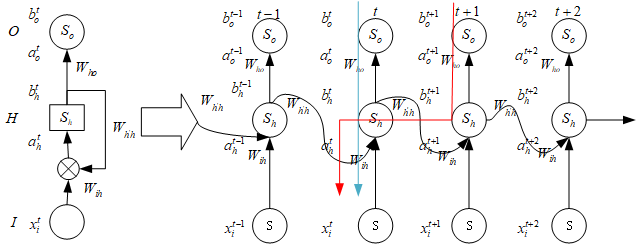RNN的结构图都是这样，按时间展开，S是隐藏层和输出层的激活函数，这里用Sigmoid，求导比较特殊，W是权重角标表示走向，O是输出层，I是输入层，H是隐藏层，a是单元的输入，b是单元的输出，上下角标是层和时间序列，x是输入层的输入，这里方便，就不给单元设置阈值了，有的代码里会有一个阈值，一般用b表示，影响不大。

神经网络的目标就是通过调整W和阈值b（不是单元的输出的b啊），来让误差变小，所谓的网络就是误差足够小的那些W和阈值b。

再上公式，简单的前向传播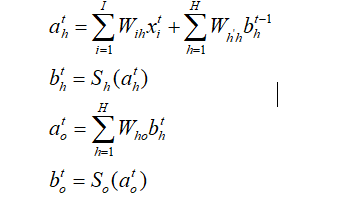再定义一个损失函数，就是上面说的优化问题的目标函数，一般为了好求导这样给出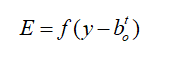这都比较简单，麻烦的是反向传播，先看简单的隐藏层到输入层的转换矩阵的所谓梯度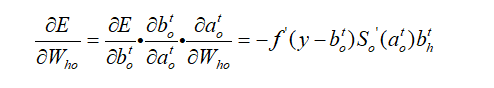接下来大的两个权重矩阵的梯度就是麻烦了，很多的文章这里就开始含糊不清，我尽量给读者写清楚，需要注意，两个矩阵的梯度都是会受到未来时刻的影响的。

首先，还是继续t时刻的蓝色线的来自输出层的反向传播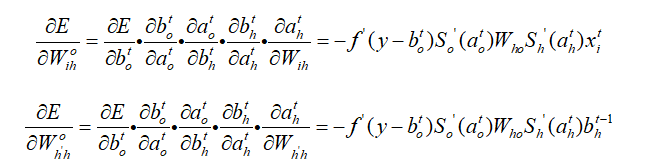接着要看红色的这条反向传播 ，需要注意，这里的t+1不单单指t+1时刻的，而是t时刻未来的所有时刻，因为t+1时刻会包含t+2时刻的信息，这一点会在之后的误差项定义中完美体现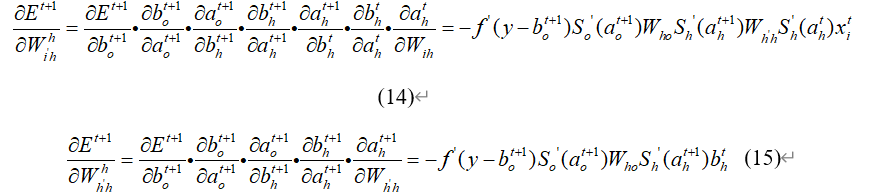两条反向传播先不着急合再一起

来看代码里的前向传播部分，先把注释删掉，文后的代码会有注释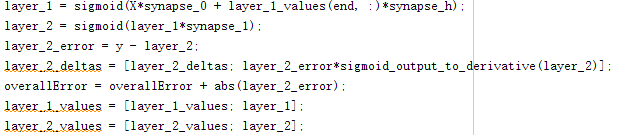里面的函数在这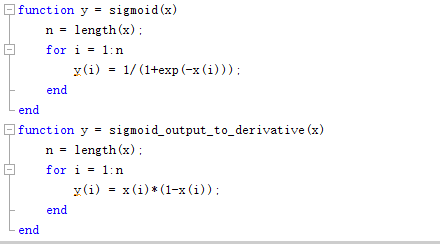麻烦的地方在第四句，其余都好懂，这个layer_2_deltas是什么鬼，再来看一下它的用处

给出反向传播和权重更新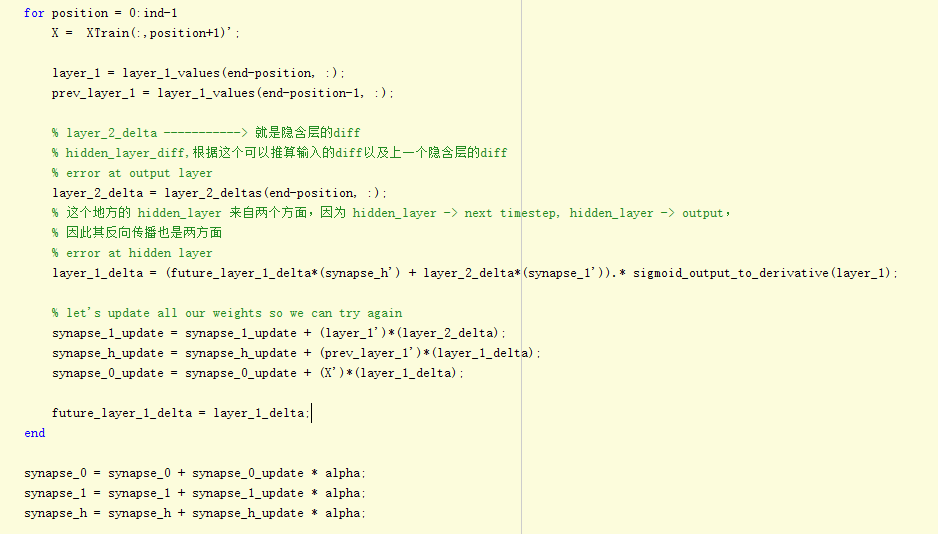下面这三行代码就是三个矩阵的更新，后面那三个加上去的就是他们对应的梯度，也就是那三个链式求导，1是隐藏层到输出层的，h是隐藏层到隐藏层的，0是输入层到隐藏层的

先分析一下这个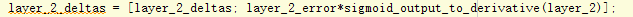包含两部分，左边的误差乘以后边的函数，前边的误差出现时因为特殊的损失函数求导后出现的，后边的函数是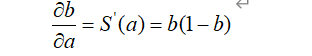这一环是链式求导里频繁出现的一环，推理确实很麻烦，直接给出结果吧

麻烦的地方在于layer_1_delta和 layer_2_delta是什么鬼，先给出结果，这两个是误差项，定义如下，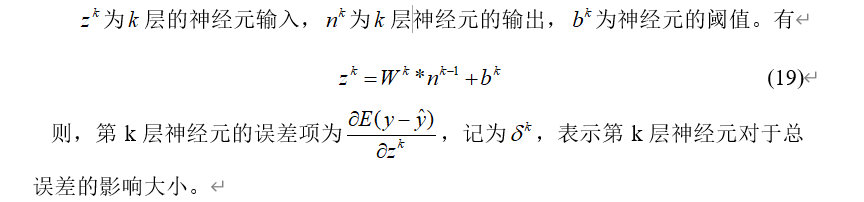误差项就是对该层的输入求偏导 ，那么代码里的layer_2_delta就是输出层的误差项，即损失函数对输出层的输入求得偏导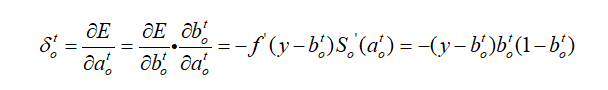那么隐藏层到输出层矩阵的梯度就可以写成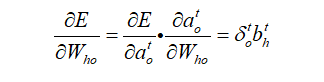接着看隐藏层的误差项layer_1_delta，即损失函数对隐藏层的输入求导，直接把两部分梯度结合起来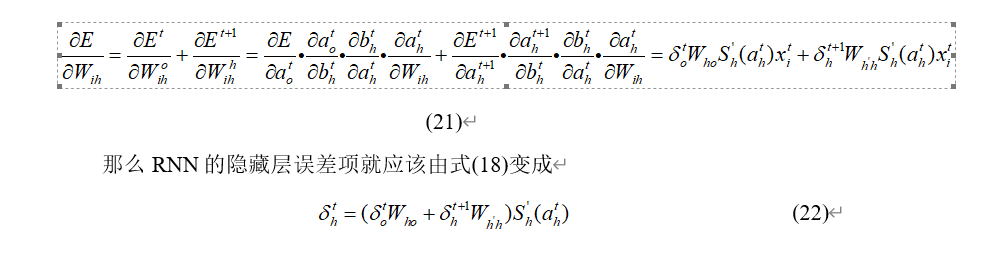需要说明的是，这个式子里包含了t+1时刻的隐藏层的误差项，代码里实现就是反向传播的计算方向和前向传播的计算方向是相反的，这样反向传播计算机会先得到下一次循环的”下一时刻“的隐藏层误差项，可以让变量保存下来。

有了 隐藏层的误差项，给最后一个矩阵求梯度就好算了算好梯度后，就可以计算权重矩阵的更新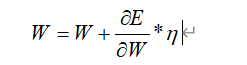后面的常数是步长，或者学习速率。

代码附上

% implementation of RNN
clc
clear
close all
%% training dataset generation
binary_dim = 8;

largest_number = 2^binary_dim - 1;
binary = cell(largest_number,1);
int2binary = cell(largest_number,1);
for i = 1:largest_number+1
binary{i} = dec2bin(i-1, 8);
int2binary{i} = binary{i};
end

%% input variables
alpha = 0.1;
input_dim = 2;
hidden_dim = 16;
output_dim = 1;

%% initialize neural network weights
synapse_0 = 2*rand(input_dim,hidden_dim) - 1;
synapse_1 = 2*rand(hidden_dim,output_dim) - 1;
synapse_h = 2*rand(hidden_dim,hidden_dim) - 1;%产生[-1,1]之间的随机数

synapse_0_update = zeros(size(synapse_0));
synapse_1_update = zeros(size(synapse_1));
synapse_h_update = zeros(size(synapse_h));

%% train logic
for j = 0:19999
% generate a simple addition problem (a + b = c)
a_int = randi(round(largest_number/2)); % int version 产生一个小于128的随机数
a = int2binary{a_int+1}; % binary encoding

b_int = randi(floor(largest_number/2)); % int version 产生一个小于127的随机数
b = int2binary{b_int+1}; % binary encoding

c_int = a_int + b_int;
c = int2binary{c_int+1};

% where we'll store our best guess (binary encoded)
d = zeros(size(c));%c的维数是1*8

if length(d)<8
pause;
end

overallError = 0;

layer_2_deltas = [];
layer_1_values = [];
layer_1_values = [layer_1_values; zeros(1, hidden_dim)];

% 开始对一个序列进行处理，搞清楚一个东西，一个LSTM单元的输出其实就是隐含层
for position = 0:binary_dim-1
X = [a(binary_dim - position)-'0' b(binary_dim - position)-'0'];   % X 是 input
y = [c(binary_dim - position)-'0']';                               % Y 是label，用来计算最后误差

% 这里是RNN，因此隐含层比较简单
% X ------------------------> input
% sunapse_0 ----------------> U_i
% layer_1_values(end, :) ---> previous hidden layer （S(t-1)）
% synapse_h ----------------> W_i
% layer_1 ------------------> new hidden layer (S(t))

layer_1 = sigmoid(X*synapse_0 + layer_1_values(end, :)*synapse_h);

% layer_1 ------------------> hidden layer (S(t))
% layer_2 ------------------> 最终的输出结果，其维度应该与 label (Y) 的维度是一致的
% 这里的 sigmoid 其实就是一个变换，将 hidden layer (size: 1 x 16) 变换为 1 x 1
% 有些时候，如果输入与输出不匹配的话，使可以使用 softmax 进行变化的
% output layer (new binary representation)
layer_2 = sigmoid(layer_1*synapse_1);

% 计算误差，根据误差进行反向传播
% layer_2_error ------------> 此次（第 position+1 次的误差）
% l 是真实结果
% layer_2 是输出结果
% layer_2_deltas 输出层的变化结果，使用了反向传播，见那个求导（输出层的输入是 layer_2，那就对输入求导即可，然后乘以误差就可以得到输出的diff）
% did we miss?... if so, by how much?
layer_2_error = y - layer_2;
layer_2_deltas = [layer_2_deltas; layer_2_error*sigmoid_output_to_derivative(layer_2)];

% 总体的误差（误差有正有负，用绝对值）
overallError = overallError + abs(layer_2_error(1));

% decode estimate so we can print it out
% 就是记录此位置的输出，用于显示结果
d(binary_dim - position) = round(layer_2(1));

% 记录下此次的隐含层 (S(t))
% store hidden layer so we can use it in the next timestep
layer_1_values = [layer_1_values; layer_1];
end

% 计算隐含层的diff，用于求参数的变化，并用来更新参数，还是每一个timestep来进行计算
future_layer_1_delta = zeros(1, hidden_dim);

% 开始进行反向传播，计算 hidden_layer 的diff，以及参数的 diff
for position = 0:binary_dim-1
% 因为是通过输入得到隐含层，因此这里还是需要用到输入的
% a -> (operation) -> y, x_diff = derivative(x) * y_diff
% 注意这里从最后开始往前推
X = [a(position+1)-'0' b(position+1)-'0'];
% layer_1 -----------------> 表示隐含层 hidden_layer (S(t))
% prev_layer_1 ------------> (S(t-1))
layer_1 = layer_1_values(end-position, :);
prev_layer_1 = layer_1_values(end-position-1, :);

% layer_2_delta -----------> 就是隐含层的diff
% hidden_layer_diff,根据这个可以推算输入的diff以及上一个隐含层的diff
% error at output layer 输出层的误差项
layer_2_delta = layer_2_deltas(end-position, :);
% 这个地方的 hidden_layer 来自两个方面，因为 hidden_layer -> next timestep, hidden_layer -> output，
% 因此其反向传播也是两方面
% error at hidden layer 隐藏层的误差项
layer_1_delta = (future_layer_1_delta*(synapse_h') + layer_2_delta*(synapse_1')).* sigmoid_output_to_derivative(layer_1);

% let's update all our weights so we can try again
synapse_1_update = synapse_1_update + (layer_1')*(layer_2_delta);
synapse_h_update = synapse_h_update + (prev_layer_1')*(layer_1_delta);
synapse_0_update = synapse_0_update + (X')*(layer_1_delta);

future_layer_1_delta = layer_1_delta;
end

synapse_0 = synapse_0 + synapse_0_update * alpha;
synapse_1 = synapse_1 + synapse_1_update * alpha;
synapse_h = synapse_h + synapse_h_update * alpha;

synapse_0_update = synapse_0_update * 0;
synapse_1_update = synapse_1_update * 0;
synapse_h_update = synapse_h_update * 0;

if(mod(j,1000) == 0)%余数
err = sprintf('Error:%s\n', num2str(overallError)); fprintf(err);
d = bin2dec(num2str(d));
pred = sprintf('Pred:%s\n',dec2bin(d,8)); fprintf(pred);
Tru = sprintf('True:%s\n', num2str(c)); fprintf(Tru);
out = 0;
size(c)
sep = sprintf('-------------\n'); fprintf(sep);
end

end
function y = sigmoid(x)
n = length(x);
for i = 1:n
y(i) = 1/(1+exp(-x(i)));
end
end
function y = sigmoid_output_to_derivative(x)
n = length(x);
for i = 1:n
y(i) = x(i)*(1-x(i));
end
end


兄弟也是第一次写，如果你看到结尾了，就给点一个赞支持一下吧，会接着这种公式加代码的讲述风格继续努力的。

展开全文深度学习 神经网络 人工智能
• 深度学习——循环神经网络RNN公式推导 1、循环神经网络引入 1.1 从传统网络到循环网络 对于传统的神经网络，在我们之前介绍的传统的神经网络中，主要包含输入层、隐藏层、输出层三个部分。其基本的图示如下： X表示...

## 深度学习——循环神经网络RNN公式推导

### 1、循环神经网络引入

#### 1.1 从传统网络到循环网络

对于传统的神经网络，在我们之前介绍的传统的神经网络中，主要包含输入层、隐藏层、输出层三个部分。其基本的图示如下：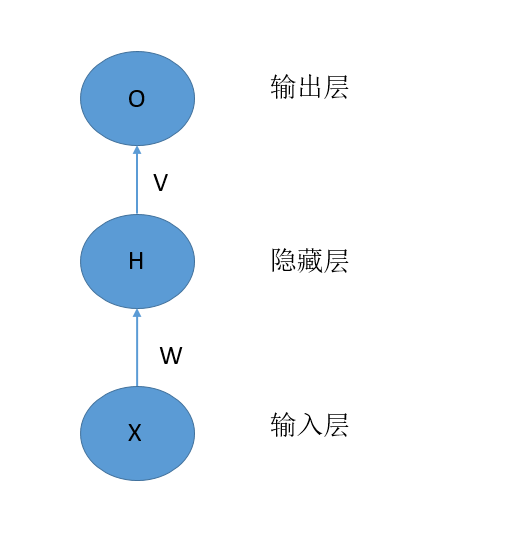X表示输入层的向量，H表示隐藏层的向量，O表示输出层的向量。W表示从输入层到隐藏层的权重矩阵，V表示从隐藏层到输出层的权重矩阵。其中，每一层可以有多个神经元。每一层神经元的个数代表的是每一层的维度。

我们将该网络展开成神经元的形式如下：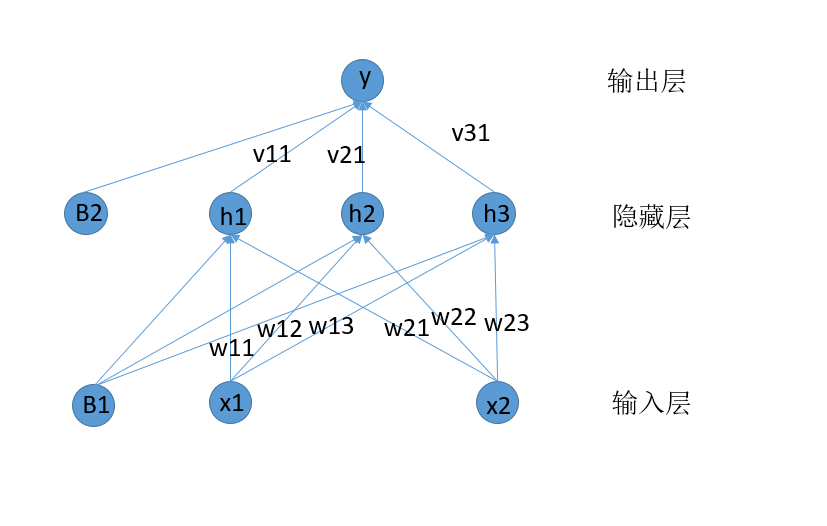我们可以看到的是，对于每一个隐藏层的神经元，其结果是由输入层的向量和权重W来决定的。似乎是有这样的一种可能，每一个隐藏层的神经元是和其排列在前面的神经元之间是有关系的，也就是排列在前面隐藏层的神经元也作为当前隐藏层神经元的一个输入。这就引出来了循环神经网络。

我们先给出循环神经网络的基本结构：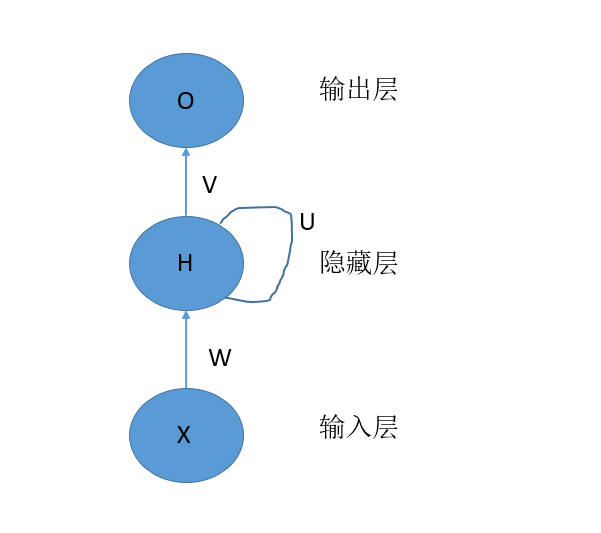在上面的结构中，我们能够发现，对于隐藏层的神经元，其中有一个自我传递信息的过程，下面是具体的展开图：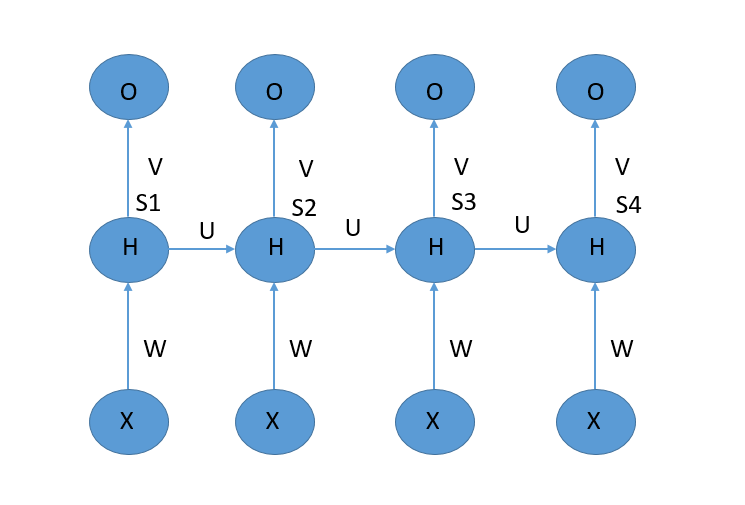可以理解为，网络的输入通过时间进行向后传播，每次输入一个X，产生一个St和一个O，然后下一个时刻的X和前一个时刻的H作为当前时刻的输入，其中X通过W来控制权重，H通过U来控制权重。我们将上述的神经网络结构成为：单向循环神经网络

在对于该神经网络进行进一步的展开(这里，我们只展开一个时刻的神经单元)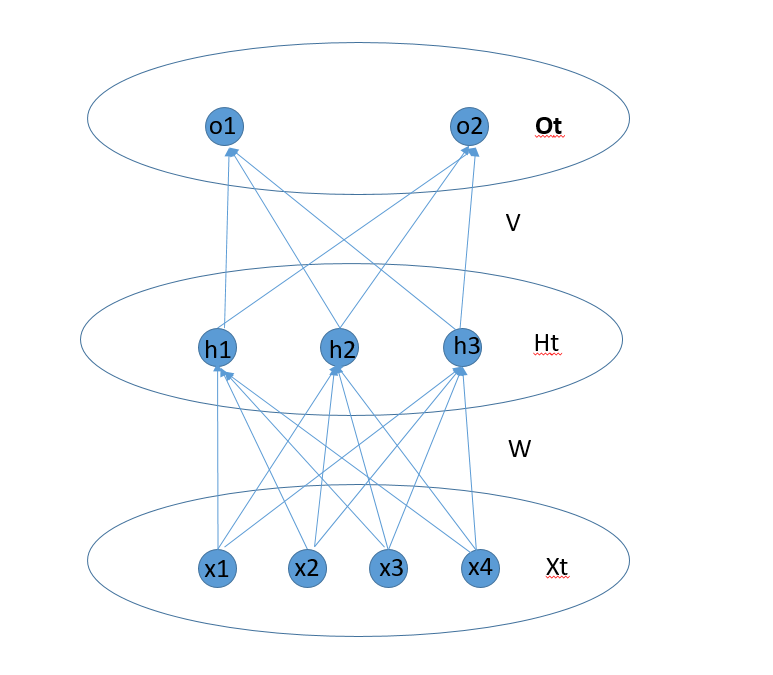### 2 BPTT算法以及前向和后向传播算法

#### 2.1 前向传播过程

1. 从输入层神经元到隐藏层神经元。
2. 从隐藏层神经元到隐藏层神经元。
3. 隐藏层神经元整合两个部分的输入。
4. 从隐藏层神经元到输出层神经元。
##### 2.1.1 从输入层神经元到隐藏层神经元

这个部分的前向传播主要是输入神经元信息和对应的权重矩阵W来决定的。具体的计算公式为：
W T X t W^TX_t
注意，这个部分中，X是一个向量，W是一个权重矩阵，通过W来将X转换成另外的向量。

##### 2.1.2 从隐藏层神经元到隐藏层神经元

这个部分主要是前一个时刻的隐藏层神经元向当前时刻的神经元的信息传递，其值有 S t − 1 S_{t-1} 和对应的权重矩阵U来决定。公式如下：
U T S t − 1 U^TS_{t-1}
注意，这个部分中， S t − 1 S_{t-1} 是一个向量，U是一个权重矩阵，通过U来将 S t − 1 S_{t-1} 转换成另外的向量。

##### 2.1.3 隐藏层神经元整合两个部分的输入

这一部分，主要是当前时刻的神经元 h t h_t ，将两个部分的输入整合，激活生成当前时刻隐藏层神经元的输出。
整合的过程就是向量相加，对应的公式为：
W T X t + U T S t − 1 W^TX_t+U^TS_{t-1}
在假设激活函数为f，则激活后生成当前时刻神经元的值：
S t = f ( W T X t + U T S t − 1 ) S_t = f(W^TX_t+U^TS_{t-1})

##### 2.1.4 从隐藏层神经元到输出层神经元

这一部分的信息传递主要是将当前时刻隐藏层神经元的值传递到当前时刻的输出神经元中去。计算公式为：
O t = g ( V T S t ) O_t = g(V^TS_t)
其中g表示的也是激活函数。

#### 2.2 前向过程总结

前向过程相对来说比较简单，用两个公式就可以总结其基本的传播过程：
S t = f ( W T X t + U T S t − 1 ) S_t = f(W^TX_t+U^TS_{t-1})
O t = g ( V T S t ) = g ( V T f ( W T X t + U T S t − 1 ) ) O_t = g(V^TS_t)=g(V^Tf(W^TX_t+U^TS_{t-1}))

#### 2.3 参数权重说明：

在整个神经网络中，一共包含的三中权重矩阵，第一个矩阵是W，给矩阵的维度为(N,K)，N表示隐藏层神经元值的向量维度，K表示输入神经单元的向量的维度。第二个权重矩阵是V，该矩阵的维度为(L,N)，其中P表示输出层的神经元的向量维度。第三个是权重矩阵U，该矩阵的维度是(N,N)。

#### 2.4 反向传播过程

##### 2.4.1 误差函数

关于误差函数的选择，可以根据具体的任务进行，我们这里选择MSE作为误差计算函数：
J = 1 2 ∑ t = 1 T ∣ ∣ R t − O t ∣ ∣ 2 J=\frac{1}{2}∑_{t=1}^T||R_t-O_t||^2
注意，我们这里在算误差的时候， R t 和 O t R_t和O_t 对应的是t时刻真实标签向量是预测输出的向量，所有采用的了取模计算的|| ||

将上面的式子展开后得到
J = 1 2 ∑ t = 1 T ∑ j = 1 L ( R t ( j ) − O t ( j ) ) 2 J = \frac{1}{2}∑_{t=1}^T∑_{j=1}^L(R_t(j)-O_t(j))^2
L表示输入向量的属性值的个数。

##### 2.4.2 反向传播过程

对于最后一个时刻的节点 X T , H T , O T X_T,H_T,O_T ，我们首先计算这一个时刻的相关误差。我们在这里设置两个临时函数：
n e t o t = V T f ( W T X t + U T S t − 1 ) neto_t = V^Tf(W^TX_t+U^TS_{t-1})
n e t h t = W T X t + U T S t − 1 neth_t = W^TX_t+U^TS_{t-1}

其中 n e t o ( t ) net_o(t) 表示t时刻没有激活的输出层的向量值， n e t h ( t ) net_h(t) 表示t时刻没有激活的隐藏层的向量值。

为了清晰，我们再之前单个神经元中的结构拿过来：根据公式和图示，我们能够知道 n e t h ( t ) neth(t) ，表示的是t时刻 [ h 1 , h 2 , h 3 ] [h_1,h_2,h_3] 组成的没有激活时的向量 n e t o ( t ) neto(t) 表示的是没有激活的 [ o 1 , o 2 ] [o_1,o_2] 构成的向量。

1. 对于最后时刻T，我们计算出来的误差是J，首先我们计算：
∂ J ∂ n e t o 1 ( T ) = ∂ J ∂ O T ( 1 ) ∂ O T ( 1 ) ∂ n e t o T ( 1 ) = ( R T ( 1 ) − O T ( 1 ) ) g ′ ( n e t o T ( 1 ) ) \frac{∂J}{∂neto_1(T)}=\frac{∂J}{∂O_T(1)}\frac{∂O_T(1)}{∂neto_T(1)}=(R_T(1)-O_T(1))g'(neto_T(1))
整理一下有：
δ T O ( j ) = ∂ J ∂ n e t o T ( j ) = ∂ J ∂ O T ( j ) ∂ O T ( j ) ∂ n e t o T ( j ) = ( R T ( j ) − O T ( j ) ) g ′ ( n e t o T ( j ) ) 其 中 j ∈ [ 1 , L ] δ_T^O(j)=\frac{∂J}{∂neto_T(j)}=\frac{∂J}{∂O_T(j)}\frac{∂O_T(j)}{∂neto_T(j)}=(R_T(j)-O_T(j))g'(neto_T(j)) 其中j∈[1,L]

进一步，转换成矩阵的形式：
δ T O = ∂ J ∂ n e t o T = ∂ J ∂ O T ∂ O T ∂ n e t o T = ( R T − O T ) ∗ g ′ ( n e t o T ) 公 式 1 δ_T^O=\frac{∂J}{∂neto_T}=\frac{∂J}{∂O_T}\frac{∂O_T}{∂neto_T}=(R_T-O_T)*g'(neto_T) 公式1

1. 然后我们计算
∂ J ∂ n e t h T ( 1 ) = ∂ J ∂ O T ( 1 ) ∂ O T ( 1 ) ∂ n e t o T ( 1 ) ∂ n e t o T ( 1 ) ∂ h T ( 1 ) ∂ h T ( 1 ) ∂ n e t h T ( 1 ) + ∂ J ∂ O T ( 2 ) ∂ O T ( 2 ) ∂ n e t o T ( 2 ) ∂ n e t o T ( 2 ) ∂ h T ( 1 ) ∂ h T ( 2 ) ∂ n e t h T ( 1 ) \frac{∂J}{∂neth_T(1)}=\frac{∂J}{∂O_T(1)}\frac{∂O_T(1)}{∂neto_T(1)}\frac{∂neto_T(1)}{∂h_T(1)}\frac{∂h_T(1)}{∂neth_T(1)}+\frac{∂J}{∂O_T(2)}\frac{∂O_T(2)}{∂neto_T(2)}\frac{∂neto_T(2)}{∂h_T(1)}\frac{∂h_T(2)}{∂neth_T(1)}

整理一下就是：
∂ J ∂ n e t h T ( 1 ) = ∑ i = 1 2 ∂ J ∂ O T ( i ) ∂ O T ( i ) ∂ n e t o T ( i ) ∂ n e t o T ( i ) ∂ h T ( 1 ) ∂ h T ( 1 ) ∂ n e t h T ( 1 ) \frac{∂J}{∂neth_T(1)}=∑_{i=1}^2\frac{∂J}{∂O_T(i)}\frac{∂O_T(i)}{∂neto_T(i)}\frac{∂neto_T(i)}{∂h_T(1)}\frac{∂h_T(1)}{∂neth_T(1)}

进一步可以推广为：
∂ J ∂ n e t h T ( i ) = ∑ i = 1 L ∂ J ∂ O T ( i ) ∂ O T ( i ) ∂ n e t o T ( i ) ∂ n e t o T ( i ) ∂ h T ( j ) ∂ h T ( j ) ∂ n e t h T ( j ) \frac{∂J}{∂neth_T(i)}=∑_{i=1}^L\frac{∂J}{∂O_T(i)}\frac{∂O_T(i)}{∂neto_T(i)}\frac{∂neto_T(i)}{∂h_T(j)}\frac{∂h_T(j)}{∂neth_T(j)}

计算出来的结果为：
δ T h ( j ) = ∂ J ∂ n e t h j ( T ) = ∑ i = 1 L δ T O ( i ) V j i f ′ ( n e t h j ( T ) ) δ_T^h(j)=\frac{∂J}{∂neth_j(T)}=∑_{i=1}^Lδ_T^O(i)V_{ji}f'(neth_j(T))

在整理成矩阵的形式：
δ T h = V T δ T O ∗ f ′ ( n e t h ( T ) ) 公 式 2 δ_T^h=V^Tδ_T^O*f'(neth(T))公式2

对于其他时刻t的关于 δ t O δ_t^O 梯度计算，也最后一个时刻T的计算方式类似：

δ t O = ∂ J ∂ n e t o t = ∂ J ∂ O t ∂ O t ∂ n e t o t = ( R t − O t ) ∗ g ′ ( n e t o t ) δ_t^O=\frac{∂J}{∂neto_t}=\frac{∂J}{∂O_t}\frac{∂O_t}{∂neto_t}=(R_t-O_t)*g'(neto_t)

注意：最重要的计算部分在于其他时刻关于 δ T h ( j ) δ_T^h(j) 的计算，下面进行具体的介绍

对于不是最后一个时刻T的 δ t h ( j ) δ_t^h(j) ，其主要包含的两个部分，一个部分是从输出层传递回来的误差，另外一个部分是从t+1时刻传递回来的误差。(因为 h t h_t 计算的结果是 h t + 1 h_{t+1} 的一个输入)

形式化描述为：
δ t h ( j ) = [ 输 出 层 误 差 + t + 1 时 刻 的 误 差 ] f ′ ( n e t h t ( j ) ) δ_t^h(j)=[输出层误差 +t+1时刻的误差]f'(neth_t(j))
在之前的计算中，我们已经知道了t时刻从输出层传递回来的误差为：
V t δ t O ∗ f ′ ( n e t h ( t ) ) V^tδ_t^O*f'(neth(t))
则有，原式为：
δ t h ( j ) = [ V t δ t O + t + 1 时 刻 的 误 差 ] f ′ ( n e t h t ( j ) ) δ_t^h(j)=[V^tδ_t^O +t+1时刻的误差]f'(neth_t(j))

下面，我们具体来计算T到T-1时刻的误差传递，根据正向传播的方式有：
S T = f ( W T X T + U T S T − 1 ) S_{T} = f(W^TX_{T}+U^TS_{T-1})
S T − 1 = f ( W T − 1 X T − 1 + U T S T − 2 ) S_{T-1}=f(W^{T-1}X_{T-1}+U^TS_{T-2})
根据上面的两个公式我们可以看出，在求 δ T − 1 h ( j ) δ_{T-1}^h(j) 的时候，需要的另外一部分是 δ T h δ T − 1 h ( j ) \frac{δ_{T}^h}{δ_{T-1}^h(j)} ,也就是
∑ i = 1 N δ T h ( i ) U j i ∑_{i=1}^Nδ_{T}^h(i)U_{ji} ，则总结上面的公式有
δ T − 1 h ( j ) = [ ∑ i = 1 L V j i δ t O ( i ) + ∑ i = 1 N δ T h ( i ) U j i ] f ′ ( n e t h T − 1 ( j ) ) δ_{T-1}^h(j)=[∑_{i=1}^LV_{ji}δ_t^O(i)+∑_{i=1}^Nδ_{T}^h(i)U_{ji}]f'(neth_{T-1}(j))
δ T − 1 h = [ V T δ T O + U T δ T h ] ∗ f ′ ( n e t h T − 1 ) δ_{T-1}^h=[V^Tδ_T^O+U^Tδ_{T}^h]*f'(neth_{T-1})

进而可以推广到其他时刻
δ t − 1 h = [ V T δ t O + U T δ t h ] ∗ f ′ ( n e t h t − 1 ) δ_{t-1}^h=[V^Tδ_t^O+U^Tδ_{t}^h]*f'(neth_{t-1})

#### 2.5 权重更新

##### 2.5.1 隐藏层到输出层V更新

V j i ( n e w ) = V j i ( o l d ) − α ∑ t = 1 T ∂ J ∂ n e t o t ( i ) ∂ n e t o t ( i ) ∂ V j i = V j i − α ∑ t = 1 T δ o t ( i ) h t ( j ) V_{ji}(new)=V_{ji}(old)-α∑_{t=1}^T\frac{∂J}{∂neto_t(i)}\frac{∂neto_t(i)}{∂V_{ji}}=V_{ji}-α∑_{t=1}^Tδ_o^t(i)h_t(j)
整理成矩阵的形式：
V ( n e w ) = V ( o l d ) + α ∑ t = 1 T δ o t h t T V(new)=V(old)+α∑_{t=1}^Tδ_o^th_t^T
注意： h t T h_t^T 中的T表示转置

##### 2.5.2 输入层到隐藏层的W更新

W j i ( n e w ) = W j i ( o l d ) − α ∑ t − 1 T ∂ J ∂ n e t h t ( i ) ∂ n e t h t ( i ) ∂ W j i = W j i ( o l d ) − α ∑ t − 1 T δ h t ( i ) X t ( j ) W_{ji}(new)=W_{ji}(old)-α∑_{t-1}^{T}\frac{∂J}{∂neth_t(i)}\frac{∂neth_t(i)}{∂W_{ji}}=W_{ji}(old)-α∑_{t-1}^{T}δ_h^t(i)X_t(j)
整理成矩阵的形式
W ( n e w ) = W ( o l d ) + α ∑ t = 1 T δ h t X t T W(new)=W(old)+α∑_{t=1}^Tδ_h^tX_t^T
注意： X t T X_t^T 中的T表示转置添加链接描述

##### 2.5.3 隐藏层到隐藏层的U的更新

U j i ( n e w ) = U j i ( o l d ) − α ∑ t − 1 T ∂ J ∂ n e t h t ( i ) ∂ n e t h t ( i ) ∂ U j i = U j i ( o l d ) − α ∑ t − 1 T δ h t ( i ) h t − 1 ( j ) U_{ji}(new)=U_{ji}(old)-α∑_{t-1}^{T}\frac{∂J}{∂neth_t(i)}\frac{∂neth_t(i)}{∂U_{ji}}=U_{ji}(old)-α∑_{t-1}^{T}δ_h^t(i)h_{t-1}(j)
整理成矩阵的形式：
U ( n e w ) = U ( o l d ) + α ∑ t = 1 T δ o t h t − 1 T U(new)=U(old)+α∑_{t=1}^Tδ_o^th_{t-1}^T
注意： h t − 1 T h_{t-1}^T 中的T表示转置

#### 3、参考资料

1. 知乎——RNN前向传播与后向传播公式推导
展开全文• ## RNN公式推导

千次阅读 2017-12-03 11:10:04
循环神经网络 神经网络是由一层一层的神经元首尾相连构成，通常情况下网络分为输入层、隐层和输出层三种。而这里要讲的循环神经网络，是下面这样一种结构：  ...话不多说，接下来我们直接开始公式

# 循环神经网络

神经网络是由一层一层的神经元首尾相连构成，通常情况下网络分为输入层、隐层和输出层三种。而这里要讲的循环神经网络，是下面这样一种结构：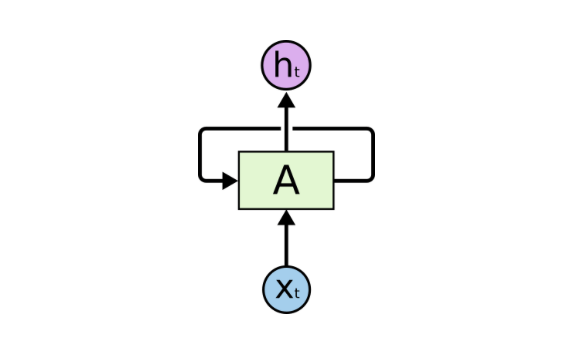如上图所示RNN结构，其隐层除了传递信息给输出层，还会传给自己，换句话说，就是传给下一时刻的隐层。这样做的目的在于，让神经网络在时间维度上产生“记忆”。至于RNN的应用场景，可自行查资料了解。话不多说，接下来我们直接开始公式推导过程。

为了便于直观理解推导，我们先按时间维度展开RNN的网络结构，并对各个部分给出相应的符号标注，如下图所示：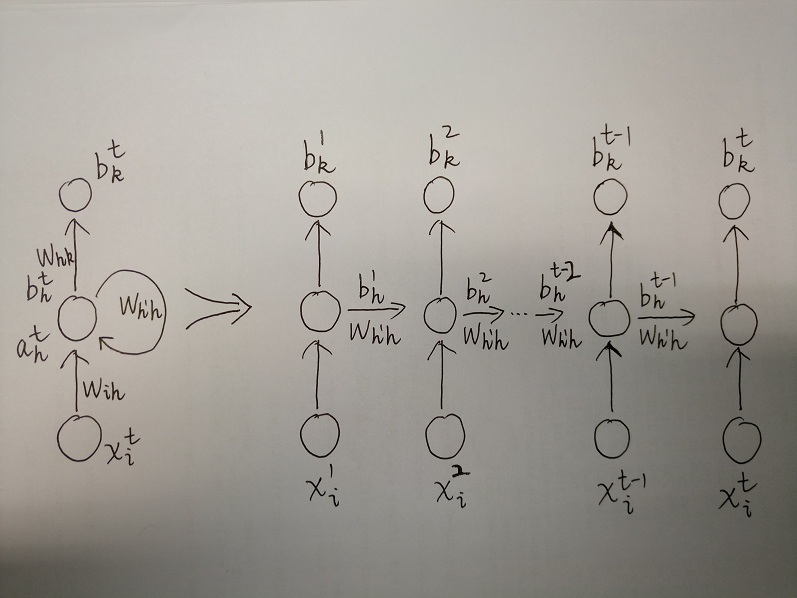其中x代表输入，W代表权重；a表示汇集计算的结果，b表示经过激活函数的结果；i是输入向量下标，h是隐层神经元编号，k是输出层神经元编号；t表示时间步。可知朴素RNN有三个权重矩阵：输入层到隐层的矩阵 Wih ，隐层到自身的矩阵 Wh’h ，以及隐层到输出层的矩阵 Whk

首先，我们写出RNN的前向传播公式。前向传播指的是从前往后传递信息，传递的是输入x。前向传播公式如下：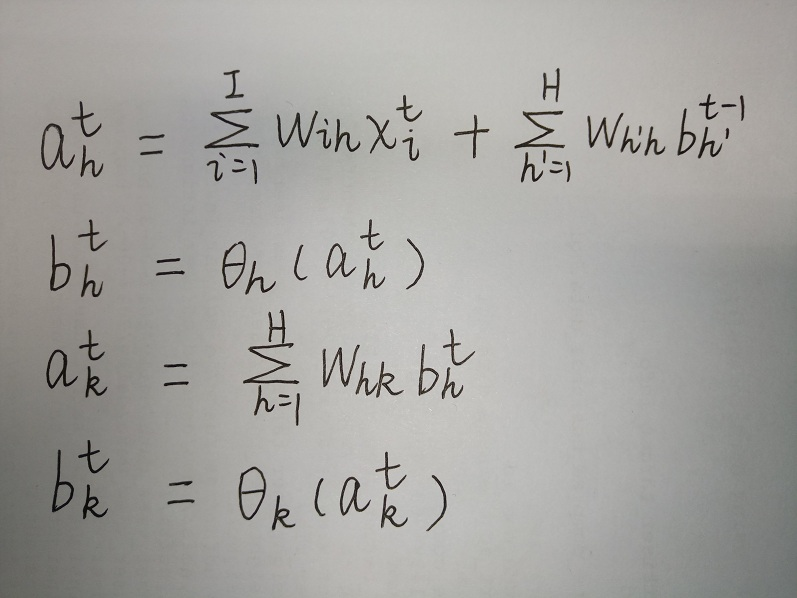上面分别描述了t时刻的隐层汇集计算（来自输入层与t-1时刻的隐层）、隐层激活输出、输出层汇集计算、输出层激活输出。

模型训练过程大致是这样：样本信息通过输入层传递到输出层，得到实际输出值，然后与真实值通过损失函数（均方差或者交叉熵公式等）计算出误差L，再反向传播分别修正三个W权重矩阵，直到误差收敛至某一阈值。反向传播指的是从后往前传递信息，传递的是误差信号L，求导之后称之为残差δ。

和普通DNN一样，根据前向传播公式，采用链式法则，我们可以很容易的给出WhkWih这两个权重矩阵的梯度计算过程以及权重更新方式，如下图所示：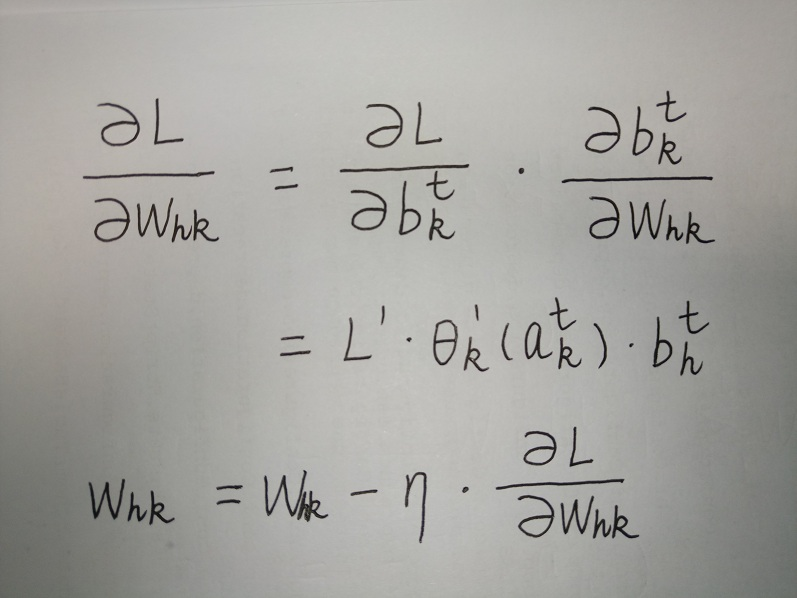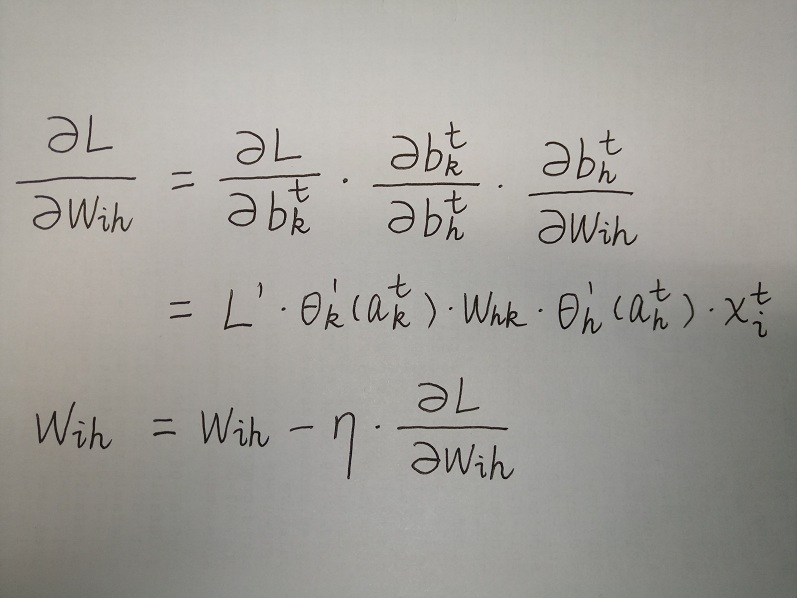其中η是学习步长， 请注意，本文给出的公式均省略了关于时间t的求和运算Σ。

RNN中比较难理解的是对Wh’h的梯度计算，因为Wh’h残差不仅来自于当前t时刻的输出层，也来自于下一个t+1时刻隐层。其中来自当前输出层的残差比较容易给出，如下图所示：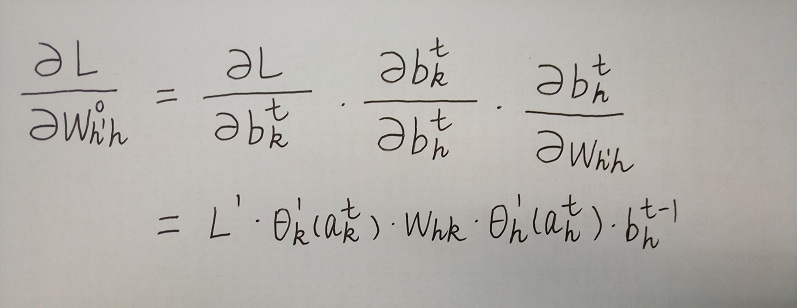而t+1时刻隐层传回的残差无法从刚才的前向传播式子中反推出来。上周日正是因为式中的隐层残差是传给t-1时刻而不是当前t时刻，才没有推出t+1时刻的残差。所以，我们需要写一个“新”的前向传播公式，其前向传播的方向如下图红色箭头所示：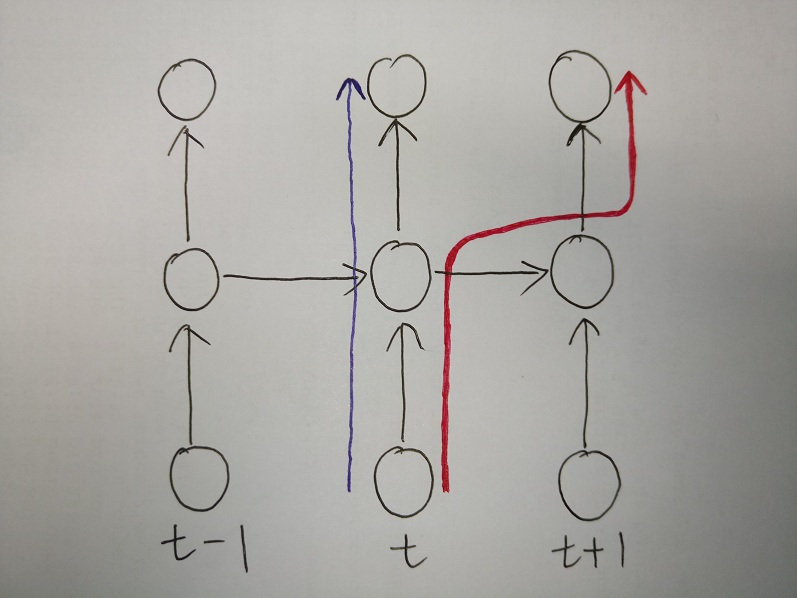针对t时刻的隐层而言，蓝色箭头的反方向代表输出层的残差传递，红色箭头的反方向则代表隐层残差传递。为了推出另一部分从t+1时刻的隐层传递回来得残差，我们不妨写出红色箭头所代表的前向传播公式：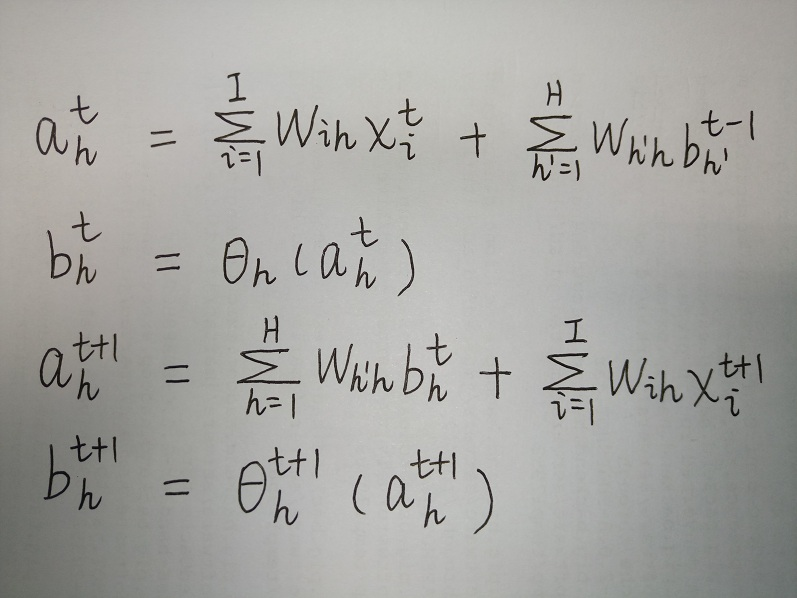跟之前的前向传播公式相比，其实就是输出层换成了下一时刻隐层。有了这个时间维度的前向传播公式，我们就能写出另一半来自t+1时刻隐层的残差公式：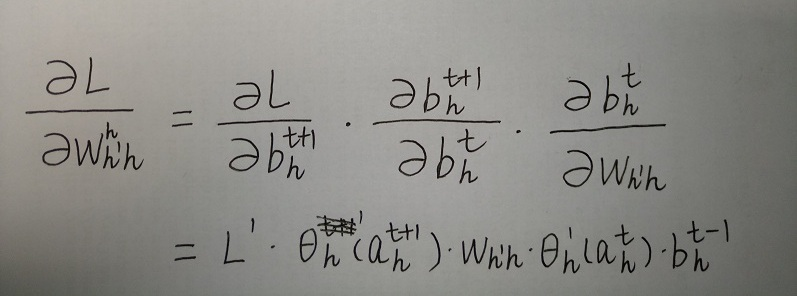最后，隐层权重向量 Wh’h 的梯度计算过程以及权重更新方式如下图所示：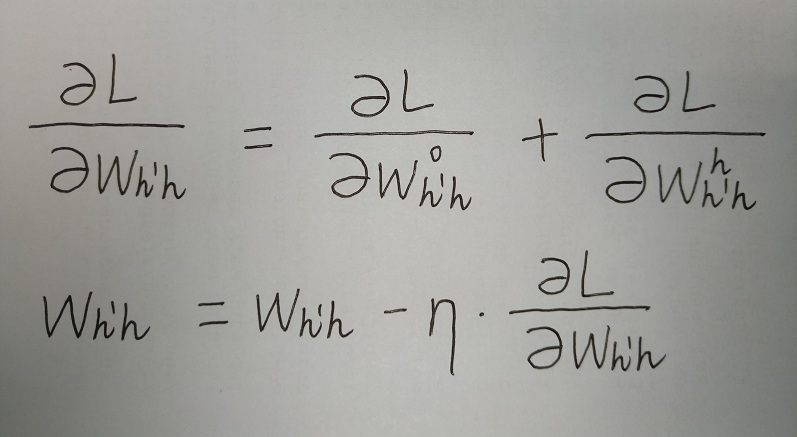至此，朴素RNN的三个权重向量W的公式就全部推导完成了。这里想特别讲一下 RNN的梯度消失问题 ，回到上面图中的那个红色箭头，这里只推导了t+1时刻隐层传回的残差。试想一下，如果是t+n时刻呢？可想而知，时间相隔越远，梯度的贡献越小。这便是朴素RNN的缺点，模型表现为容易“忘事”。但经过改进的RNN模型可以克服这个缺点，便是我们上节提到的LSTM模型。敬请期待下节。

对本文推导有任何问题或疑问，欢迎留言交流。

转载自：http://m.blog.csdn.net/cherrylvlei/article/details/75269614

展开全文• RNN中，最普通的一种LSTM，每个小RNNCell具有4个gate，即4个layer，对于同样的一个RNNCell在不同的资料上面，表达公式略有出入。 这三个layer，activation function：三个sigmoid，一个tanh。 正常的LSTM： 附上程序...
• ## RNN及其公式推导

万次阅读 2017-09-13 19:33:41
RNN及其公式推导 RNN即循环神经网络，循环神经网络的种类有很多，本文主要是对基本的神经网络进行推导。一开始对推导很晕，在阅读了许多资料之后，整理如下。 结构
• 一、循环神经网络（RNN）原理通俗解释 在图像处理中，目前做的最好的是CNN 自然语言处理中，表现比较好的是RNN 既然我们已经有了人工神经网络和卷积神经网络，为什么还要循环神经网络？ 原因很简单，无论是卷积...人工智能 深度学习
• rnn = RNN(vocab_size, 2) def train(data, backprop=True): items = list(data.items()) random.shuffle(items) loss = 0 num_correct = 0 for x, y in items: inputs = text_to_array(x) target = int...python
• 如果用RNN来预测Teddy是不是人名，它只会根据这三个单词（He, said, Teddy）来进行预测，而这3个单词是无法预测准确的。 要准确预测，就必须考虑Teddy之后的信息，而传统的RNN并不会考虑之后的信息。（后面会讲到...
• 最近工作中用到了循环神经网络（Recurrent Neural Networks），感觉网上的...因此于周日闲暇时光试推公式，却陷于时间维度的反向传播推导之中，直到昨晚才恍然大悟。在这里与大家分享我的推导，也便于日后温习与查阅。机器学习 神经网络 循环神经网络 公式推导
• 循环神经网络（recurrent neural network, RNN）源自1982年由Saratha Sathasivam提出的霍普菲尔德网络。霍普菲尔德网络因为实现困难，在提出时并且没有被合适地应用。该网络结构也于1986年后被全连接神经网络以及...循环神经网络
• RNN 定义模型 ht是上一层传过来的隐藏层,x是特征矩阵，w为正太分布矩阵均值为零方差为0.01h_t是上一层传过来的隐藏层, x是特征矩阵，w为正太分布矩阵 均值为零方差为0.01ht​是上一层传过来的隐藏层,x是特征矩阵，w...pytorch 深度学习
• 最近在整理tensorflow，经常用到RNN与lSTM，故整理如下： -RNN：循环神经网络(Recurrent Neural Networks) -LSTM：长短时记忆网络（Long Short-Term Memory） 在看这篇文章之前，如果之前没有接触过-神经网络，请...RNN与lstm tensorflow 循环神经网络原理
•RNN 深度学习
• RNN结构如图所示： Xt∈RxX^t\in R^x表示t时刻的输入(XtX^t是多少维，则这一层有多少个神经元，这里设为xx维，图中画的是3维）ht∈Rhh^t\in R^h表示t时刻隐层的输出(假设这一层有h个神经元）yt∈Ryy^t\in R^y表示t...
• RNN网络 RNN模型计算分为两步，第一步，计算第ｔ个时间步的隐藏层ａ；第二步，计算t步的预测值ｙ。 其中Wax和Waa两组参数分别与前一层的激活a和当前数据x结合，也可将其合二为一，并与x和a的连接计算...公式中的Γ
• RNN 公式： ht=f(W⋅[ht−1,xt]+b)h_{t}=f\left(W \cdot\left[h_{t-1}, x_{t}\right]+b\right)ht​=f(W⋅[ht−1​,xt​]+b) LSTM 公式： 遗忘门：ft=σ(Wf⋅[ht−1,xt]+bf)f_{t}=\sigma\left(W_{f} \cdot\left[h_{...深度学习 lstm 神经网络 nlp
• 文章目录RNN标准RNN代码 RNN 标准RNN 在PyTorch中的调用也非常简单，使用 nn.RNN（）即可调用，下面依次介绍其中的参数。 RNN() 里面的参数有 input_size 表示输入 xt 的特征维度 hidden_size 表示输出的特征维度 ...
• 作者主页(文火冰糖的硅基工坊)：文火冰糖（王文兵）的博客_文火冰糖的硅基工坊_CSDN博客 ...第2章 循环神经网络RNN的结构 - 个人理解 2.1 “非时序”的组合单元的网络结构 2.2RNN基本的结构：“时序”单元 2..pytorch 循环神经网络
• RNN 反向传播公式推导（非矩阵式） 因为模式识别非要用Latex来编辑公式，所以逼着我写一次博客。嗯，说不定这是一个很好的开始。因为笔者的水平有限，所以如果有不慎有疏漏，烦请不吝赐教。 参考的博客是： 循环神经...
• 本文是全系列中第5 / 7篇：Keras 从入门到精通 使用Keras进行深度学习：（一）Keras 入门 使用Keras进行深度学习：（二）CNN讲解及实践 ... 使用Keras进行深度学习：（五）RNN和双向RNN讲解及实践 使用Keras进...
• 好久没用正儿八经地写博客了，csdn居然也有了markdown的编辑器了，最近花了不少时间看RNN以及LSTM的论文，在组内『夜校』分享过了，再在这里总结一下发出来吧，按照我讲解的思路，理解RNN以及LSTM的算法流程并推导一...
• 转载自：https://zhuanlan.zhihu.com/p/28806793Recurrent Neural Network(RNN)...在隐层上增加了一个反馈连接，也就是说，RNN隐层当前时刻的输入有一部分是前一时刻的隐层输出，这使得RNN可以通过循环反馈连接保留...
• 注：本文主要扣反向传播这一块的公式rnn原理、历史发展等等其他文章都讲的很清楚了 前向传播公式 nett=Uxt+Wht−1+bnet^t= Ux^t +Wh^{t-1} +bnett=Uxt+Wht−1+b ht=tanh(nett)h^t = tanh(net^t)ht=tanh(nett) ot=...python jupyter 循环神经网络
• 上图是经典的双向RNN模型，我们知道该模型是通过递归的方式运行，虽然适合对序列数据建模，但是缺点也很明显“它无法并行执行”也就无法利用GPU强大的并行能力（这里插句题外话，正因为GPU强大的并行能力，所以batch...
• BP的流程： CNN 前向： 反向： 尺寸计算 参数计算 RNN 前向： 后向：...